## RS Aggarwal Class 8 Solutions Chapter 4 Cubes and Cube Roots Ex 4C

These Solutions are part of RS Aggarwal Solutions Class 8. Here we have given RS Aggarwal Solutions Class 8 Chapter 4 Cubes and Cube Roots Ex 4C.

Other Exercises

Evaluate:

Question 1.
Solution:
$$\sqrt [ 3 ]{ 64 }$$
= $$\sqrt [ 3 ]{ 4X4X4 }$$
= $$\sqrt [ 3 ]{ { 4 }^{ 3 } }$$
= 4

Question 2.
Solution:
$$\sqrt [ 3 ]{ 343 }$$
= $$\sqrt [ 3 ]{ 7X7X7 }$$
= $$\sqrt [ 3 ]{ { 7 }^{ 3 } }$$
= 7

Question 3.
Solution:
$$\sqrt [ 3 ]{ 729 }$$Question 4.
Solution:
$$\sqrt [ 3 ]{ 1728 }$$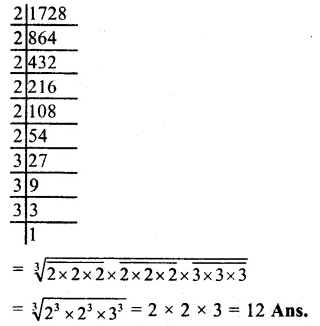Question 5.
Solution:
$$\sqrt [ 3 ]{ 9261 }$$Question 6.
Solution:
$$\sqrt [ 3 ]{ 4096 }$$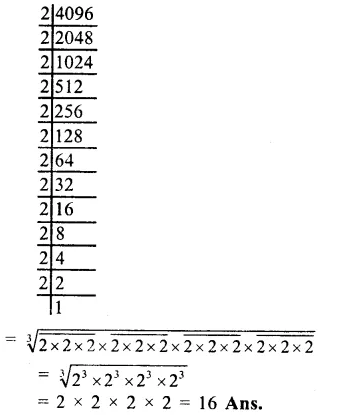Question 7.
Solution:
$$\sqrt [ 3 ]{ 8000 }$$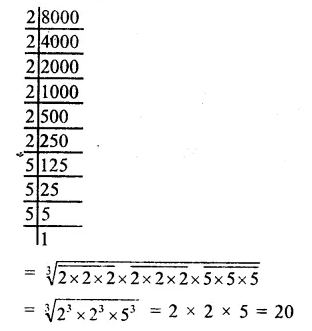Question 8.
Solution:
$$\sqrt [ 3 ]{ 3375 }$$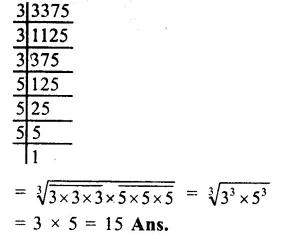Question 9.
Solution:
$$\sqrt [ 3 ]{ -216 }$$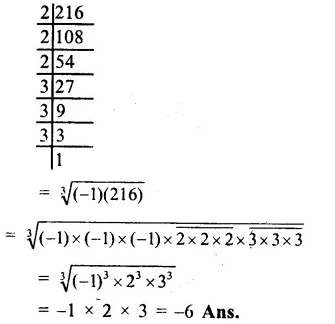Question 10.
Solution:
$$\sqrt [ 3 ]{ -512 }$$Question 11.
Solution:
$$\sqrt [ 3 ]{ -1331 }$$Question 12.
Solution:
$$\sqrt [ 3 ]{ \frac { 27 }{ 64 } }$$Question 13.
Solution:
$$\sqrt [ 3 ]{ \frac { 125 }{ 216 } }$$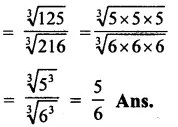Question 14.
Solution:
$$\sqrt [ 3 ]{ \frac { -27 }{ 125 } }$$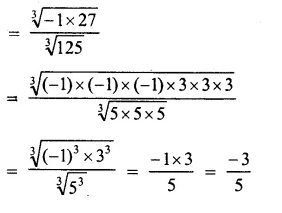Question 15.
Solution:
$$\sqrt [ 3 ]{ \frac { -64 }{ 343 } }$$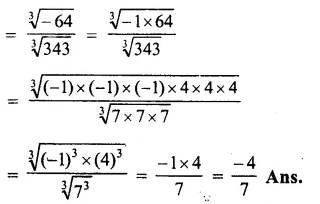Question 16.
Solution:
$$\sqrt [ 3 ]{ 64\times 729 }$$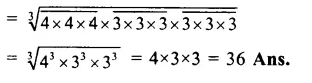Question 17.
Solution:
$$\sqrt [ 3 ]{ \frac { 729 }{ 1000 } }$$Question 18.
Solution:
$$\sqrt [ 3 ]{ \frac { -512 }{ 343 } }$$Hope given RS Aggarwal Solutions Class 8 Chapter 4 Cubes and Cube Roots Ex 4C are helpful to complete your math homework.

If you have any doubts, please comment below. Learn Insta try to provide online math tutoring for you.